Valgrind *不是* 泄漏检查工具已翻译 100%

oschina 投递于 2014/12/09 07:26 (共 10 段, 翻译完成于 12-11)

概要:

valgrind ./myProgram myProgramsFirstArg myProgramsSecondArg

1) 误用未初始化的值. 这也是它的基本功：

bool condition;
if (condition) {
//Do the thing
}

==2364== Conditional jump or move depends on uninitialized value(s)
==2364==    at 0x400916: main (test.cpp:106)

bool condition;
if (foo) {
condition = true;
}
if (bar) {
condition = false;
}
if (baz) {
condition = true;
}
if (condition) {
//Do the thing
}

2) 操作你不该去碰的内存。读写从来没被分配出来的内存，被释放掉的内存;访问超过一块分配好的内存的边界的内存;栈上不能读写的内存。

vector<int> v { 1, 2, 3, 4, 5 };
v = 0; //Oops

==2710== Invalid write of size 4
==2710==    at 0x400961: foo() (test.cpp:85)
==2710==    by 0x4009A2: main (test.cpp:89)
==2710==  Address 0x5a1d054 is 0 bytes after a block of size 20 alloc'd
==2710==    at 0x4C2B0E0: operator new(unsigned long) (in /usr/lib/valgrind/vgpreload_memcheck-amd64-linux.so)
==2710==    by 0x400EDF: __gnu_cxx::new_allocator<int>::allocate(unsigned long, void const*) (new_allocator.h:104)
==2710==    by 0x400DCE: std::_Vector_base<int, std::allocator<int> >::_M_allocate(unsigned long) (in /home/mark/test/a.out)
==2710==    by 0x400C5F: void std::vector<int, std::allocator<int> >::_M_range_initialize<int const*>(int const*, int const*, std::forward_iterator_tag) (stl_vector.h:1201)
==2710==    by 0x400AF4: std::vector<int, std::allocator<int> >::vector(std::initializer_list<int>, std::allocator<int> const&) (stl_vector.h:368)
==2710==    by 0x400943: foo() (test.cpp:84)
==2710==    by 0x4009A2: main (test.cpp:89)

3) 误用 std::memcpy 以及基于该函数构建的其他函数会导致你的源数组和目标数组地址重叠 （请先 阅读我的这篇文章 里面解释了为什么 std::memcpy 会被弃用，并牢记当你使用其他看似不错的更高层级的抽象层时，你依然无法避免间接的调用到 std::memcpy）

4) 无效的内存释放 （在现代代码中已经几乎没有了，总之您应该优先使用智能指针）

5) 数据竞争：

valgrind --tool=helgrind ./myProgram

auto x = 0;
++x;
}).detach();
++x;

==2872== Possible data race during read of size 4 at 0xFFEFFFE8C by thread #1
==2872== Locks held: none
==2872==    at 0x401081: main (test.cpp:96)
==2872==
==2872== This conflicts with a previous write of size 4 by thread #2
==2872== Locks held: none
==2872==    at 0x40103A: main::{lambda()#1}::operator()() const (test.cpp:94)
==2872==    by 0x401F2D: void std::_Bind_simple<main::{lambda()#1} ()>::_M_invoke
<>(std::_Index_tuple<>) (functional:1732)
==2872==    by 0x401E84: std::_Bind_simple<main::{lambda()#1} ()>::operator()()
(functional:1720)
==2872==    by 0x401E1D: std::thread::_Impl<std::_Bind_simple<main::{lambda()#1}
==2872==    by 0x4EEEBEF: ??? (in /usr/lib/x86_64-linux-gnu/libstdc++.so.6.0.19)
==2872==    by 0x4C30E26: ??? (in /usr/lib/valgrind/vgpreload_helgrind-amd64-linu
x.so)
==2872==    by 0x566FEFC: clone (clone.S:111)

6) 欧耶... 内存泄露了, 你不会还没启用智能指针吧。

valgrind --leak-check=full ./myProgram

（如果你忘了是哪个参数的话，只要像往常那样运行一次 Valgrind，它会在输出中的内存摘要部分提醒你的）

auto x = new int(5);

Valgrind 会有如下输出：

==2881== 4 bytes in 1 blocks are definitely lost in loss record 1 of 1
==2881==    at 0x4C2B0E0: operator new(unsigned long) (in /usr/lib/valgrind/vgp
==2881==    by 0x400966: main (test.cpp:92)

Valgrind 用作函数和内存分析:

Valgrind 不仅能告诉您错误出在哪里，他还能帮您优化代码。 人们常常自以为知道是什么导致了程序在运行时大量消耗内存...，一番折腾之后才发现错了。 怎样才能节约您宝贵的时间呢? 多测量。

valgrind --tool=callgrind ./myProgram

Valgrind 远不止是一款内存泄露检测工具。是时候改变您的观念了： Valgrind 要做未知行为的清道夫。

Valgrind 完全可以作为您的首选工具。他不仅向您报告错误的地点和原因，关键是他会抢在程序奔溃之前提醒您（这两点都是 GDB 无法做到的）。 当然 GDB 依然优秀，它能在断言失败时给出完整详尽的堆栈跟踪信息，这对调试并发代码和其他一些情况都是很必要的。

-pedantic -Wall -Wextra 这些编译选项也是相当有用的。越来越聪明的现代编译器也能帮你定位一些未知行为。Valgrind 应该被当做对编译器的有力的补足，而非功能重叠的竞争者。

Address Sanitizer for clang and g++
Undefined Behavior Sanitizer for clang and g++
Memory Sanitizer for clang
Thread Sanitizer for clang

评论(14)

y
mark,学习了，非常不错
a

引用来自“timxx”的评论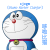0.0 我读书少，不要骗我

引用来自“eechen”的评论

Markdown里在文字两侧加星号表示强调。

引用来自“Raphael_goh”的评论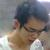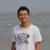valgrind思路还是不错的，但是有点太重量级。没记错的话，它是弄了个类似虚拟CPU之类的玩意，你原本的程序有一个字节，它也对应生成一段数据。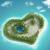0.0 我读书少，不要骗我

引用来自“eechen”的评论

Markdown里在文字两侧加星号表示强调。

引用来自“月影南溪”的评论0.0 我读书少，不要骗我

引用来自“eechen”的评论

Markdown里在文字两侧加星号表示强调。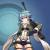0.0 我读书少，不要骗我

引用来自“eechen”的评论

Markdown里在文字两侧加星号表示强调。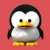Qt Creator 和 Eclipse CDT 里都继承了 Valgrind 和 GDB 前端：
http://my.oschina.net/eechen/blog/166969引用来自“月影南溪”的评论

0.0 我读书少，不要骗我
Markdown里在文字两侧加星号表示强调。